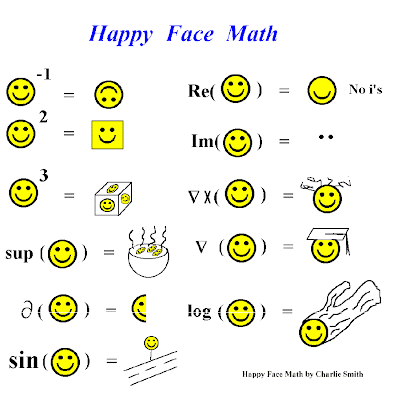## Tuesday, July 19, 2011

### International Math Olympiad 2011

When I participated at IMO 1992 in Moscow, people were laughing that our year was so unlucky to have Moscow - but I am actually happy it was Moscow.

This year, kids compete in Amsterdam which I visited in 1997 and 2002, to make you start to think about functions with period 5 which you will actually find helpful momentarily. ;-)Terence Tao will probably ask his readers to solve the most difficult problem, Q6. Phil Gibbs is modest so he chose Q1, maybe the easiest one.

As you know, I am even more modest, so let's start with a special problem for Miss USA participants of the IMO 2011:A nice solution. Well, it was too easy. Here is a well-known problem for the climate alarmists:You may prefer some problems involving smiling faces:Via Tetrahedral where you may find additional jokes.

But back to IMO 2011.

Here are all the six problems and those from the previous years. Let me choose Q5 which looks easy:
Q5: Let $$f$$ be a function from the set of integers to the set of positive integers. Suppose that, for any two integers $$m$$ and $$n$$, the difference $$f(m)-f(n)$$ is divisible by $$f(m-n)$$. Prove that, for all integers $$m$$ and $$n$$ with $$f(m)\leq f(n)$$, the number $$f(n)$$ is divisible by $$f(m)$$.
If you can solve it, boast in the comments.

Pilsner soccer rules

Czech top soccer league's champion, Victoria Pilsner, had defeated Pyunik Yerevan in the Champions League when it played in Armenia, 4-to-0. But what about the second match? Some people were afraid. I wasn't. A mile away from my home, on the "Pilsner construction site", our team smashed Yerevan 5-to-1, to make the aggregate score 9-to-1.

#### 6 comments:

1.You see, a nice task without real numbers. You want to learn them real numbers and the majority of people can't solve tasks with integers. What is leq?
I probably can't prove it, I am weak in algebra. :P

2.Disappointing to hear that your web page didn't get converted to LaTeX. Did you turn off JavaScript? Or what browser/OS do you use?

\leq appears as $$\leq$$ and it means "less than or equal to".

3.Let f(m)=2^(2m) when m is a positive integer and f(m)=2^(-2m-1) when m is a negative integer.

4.Amsterdam dam in 2002 ?

really ? don't remember the LMoth face in 2002...nope

5.I use NoScript and my javascript was partialy disabled. Thanks.

6.This is a humble try and I could be wrong.
Apologies that I don't know how to use the Mathjax.

----

The problem can be re-stated
Given k.f(m) = f(n)
prove than k is an element of Z+

----

f(m) - f(n) = j.f(m - n), where j is an element of Z.

j can be expressed as a function,

f(m) - f(n) = j(m, n).f(m - n), where j(m, n) is an element of Z.

For convenience, this can be re-stated,

f(n) - f(m) = [-j(m, n)].f(m - n)

f(n) - f(m) = p(m, n).f(m - n)

f(n) >= f(m) implies p(m, n) is an element of N.

----

k.f(m) - f(m) = p(m, n).f(m - n)

k = 1 + p(m, n).f(m - n) / f(m)

Therefore, k is an element of Z+ if f(m - n) | f(m)

----

Let n = 0

f(0) - f(m) = p(m, 0).f(m)

This can be generalized for any n or m,

f(0) = f(x) [1 + p(x, 0)]

Therefore,

f(0) is the maximum and is always a factor of f(n) and f(m) including f(m - n) for any n or m

f is a set of positive factors.

----

f(0) | f(m)

f(0) | f(m - n)

f(0) | [f(m - n) - f(m)]

f(0) = y.[f(m - n) - f(m)], where y is an element of Z

f(0) / f(m) = y.[f(m - n) / f(m) - 1]

f(0) | f(m) implies f(m - n) | f(m)

k is an element of Z+